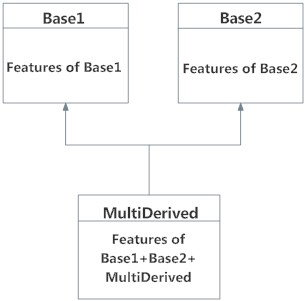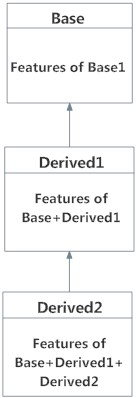# Python Multiple Inheritance

In this tutorial, you'll learn about multiple inheritance in Python and how to use it in your program. You'll also learn about multi-level inheritance and the method resolution order.

## Python Multiple Inheritance

A class can be derived from more than one base class in Python, similar to C++. This is called multiple inheritance.

In multiple inheritance, the features of all the base classes are inherited into the derived class. The syntax for multiple inheritance is similar to single inheritance.

### Example

``````class Base1:
pass

class Base2:
pass

class MultiDerived(Base1, Base2):
pass``````

Here, the MultiDerived class is derived from Base1 and Base2 classes.Multiple Inheritance in Python

The MultiDerived class inherits from both Base1 and Base2 classes.

## Python Multilevel Inheritance

We can also inherit from a derived class. This is called multilevel inheritance. It can be of any depth in Python.

In multilevel inheritance, features of the base class and the derived class are inherited into the new derived class.

An example with corresponding visualization is given below.

``````class Base:
pass

class Derived1(Base):
pass

class Derived2(Derived1):
pass``````

Here, the Derived1 class is derived from the Base class, and the Derived2 class is derived from the Derived1 class.Multilevel Inheritance in Python

## Method Resolution Order in Python

Every class in Python is derived from the `object` class. It is the most base type in Python.

So technically, all other classes, either built-in or user-defined, are derived classes and all objects are instances of the `object` class.

``````# Output: True
print(issubclass(list,object))

# Output: True
print(isinstance(5.5,object))

# Output: True
print(isinstance("Hello",object))``````

In the multiple inheritance scenario, any specified attribute is searched first in the current class. If not found, the search continues into parent classes in depth-first, left-right fashion without searching the same class twice.

So, in the above example of `MultiDerived` class the search order is [`MultiDerived`, `Base1`, `Base2`, `object`]. This order is also called linearization of `MultiDerived` class and the set of rules used to find this order is called Method Resolution Order (MRO).

MRO must prevent local precedence ordering and also provide monotonicity. It ensures that a class always appears before its parents. In case of multiple parents, the order is the same as tuples of base classes.

MRO of a class can be viewed as the `__mro__` attribute or the `mro()` method. The former returns a tuple while the latter returns a list.

``````>>> MultiDerived.__mro__
(<class '__main__.MultiDerived'>,
<class '__main__.Base1'>,
<class '__main__.Base2'>,
<class 'object'>)

>>> MultiDerived.mro()
[<class '__main__.MultiDerived'>,
<class '__main__.Base1'>,
<class '__main__.Base2'>,
<class 'object'>]``````

Here is a little more complex multiple inheritance example and its visualization along with the MRO.Visualizing Multiple Inheritance in Python
``````# Demonstration of MRO

class X:
pass

class Y:
pass

class Z:
pass

class A(X, Y):
pass

class B(Y, Z):
pass

class M(B, A, Z):
pass

# Output:
# [<class '__main__.M'>, <class '__main__.B'>,
#  <class '__main__.A'>, <class '__main__.X'>,
#  <class '__main__.Y'>, <class '__main__.Z'>,
#  <class 'object'>]

print(M.mro())``````

Output

`[<class '__main__.M'>, <class '__main__.B'>, <class '__main__.A'>, <class '__main__.X'>, <class '__main__.Y'>, <class '__main__.Z'>, <class 'object'>]`

To know the actual algorithm on how MRO is calculated, visit Discussion on MRO.